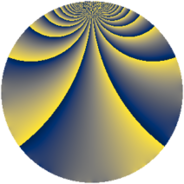# Properties

 Label 2415.2.zLevel $2415$ Weight $2$ Character orbit 2415.z Rep. character $\chi_{2415}(1724,\cdot)$ Character field $\Q(\zeta_{6})$ Dimension $752$ Sturm bound $768$

# Related objects

## Defining parameters

 Level: $$N$$ $$=$$ $$2415 = 3 \cdot 5 \cdot 7 \cdot 23$$ Weight: $$k$$ $$=$$ $$2$$ Character orbit: $$[\chi]$$ $$=$$ 2415.z (of order $$6$$ and degree $$2$$) Character conductor: $$\operatorname{cond}(\chi)$$ $$=$$ $$2415$$ Character field: $$\Q(\zeta_{6})$$ Sturm bound: $$768$$

## Dimensions

The following table gives the dimensions of various subspaces of $$M_{2}(2415, [\chi])$$.

Total New Old
Modular forms 784 784 0
Cusp forms 752 752 0
Eisenstein series 32 32 0

## Trace form

 $$752q - 376q^{4} - 8q^{6} - 8q^{9} + O(q^{10})$$ $$752q - 376q^{4} - 8q^{6} - 8q^{9} - 376q^{16} - 4q^{24} + 4q^{25} - 8q^{31} + 56q^{36} + 24q^{39} - 28q^{46} - 8q^{49} - 20q^{54} - 40q^{55} + 704q^{64} + 52q^{69} + 76q^{70} - 50q^{75} + 32q^{81} - 160q^{85} - 8q^{94} + 16q^{96} + O(q^{100})$$

## Decomposition of $$S_{2}^{\mathrm{new}}(2415, [\chi])$$ into newform subspaces

The newforms in this space have not yet been added to the LMFDB.